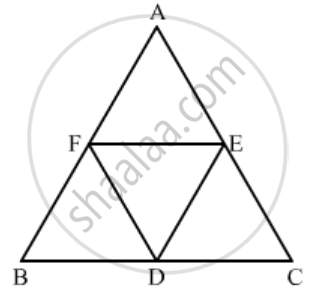Advertisement Remove all ads

# If D, E, F Are the Mid-points of Sides Bc, Ca and Ab Respectively of ∆Abc, Then the Ratio of the Areas of Triangles Def and Abc is (A) 1 : 4 (B) 1 : 2 (C) 2 : 3 (D) 4 : 5 - Mathematics

MCQ

If D, E, F are the mid-points of sides BC, CA and AB respectively of ∆ABC, then the ratio of the areas of triangles DEF and ABC is

#### Options

• 1 : 4

• 1 : 2

• 2 : 3

• 4 : 5

Advertisement Remove all ads

#### Solution

GIVEN: In ΔABC, D, E and F are the midpoints of BC, CA, and AB respectively.

TO FIND: Ratio of the areas of ΔDEF and ΔABC

Since it is given that D and, E are the midpoints of BC, and AC respectively.

Therefore DE || AB, DE || FA……(1)

Again it is given that D and, F are the midpoints of BC, and, AB respectively.

Therefore, DF || CA, DF || AE……(2)

From (1) and (2) we get AFDE is a parallelogram.

Similarly we can prove that BDEF is a parallelogram.

Now, in ΔADE and ΔABC

∠FDE=∠A\text{(opposite angles of}||^(gm)AFDE)

∠DEF = ∠ B=\text{(opposite angles of}||^(gm)BDEF)

⇒ Δ ABC ∼ Δ DEF (\text{AA similarity criterion})We know that the ratio of areas of two similar triangles is equal to the ratio of squares of their corresponding sides.

{ar(Δ DEF)}/{ar(Δ ABC)}=((DE)/(AB))^2

{ar(Δ DEF)}/{ar(Δ ABC)}=((1/2(AB))/(AB))^2(Since DE =1/2AB)

{ar(Δ DEF)}/{ar(Δ ABC)}=(1/4)

Hence the correct option is a

Concept: Triangles Examples and Solutions
Is there an error in this question or solution?
Advertisement Remove all ads

#### APPEARS IN

RD Sharma Class 10 Maths
Chapter 7 Triangles
Q 12 | Page 132
Advertisement Remove all ads

#### Video TutorialsVIEW ALL 

Advertisement Remove all ads
Share
Notifications

View all notifications

Forgot password?
Course